Test: Set Theory & Algebra- 1

# Test: Set Theory & Algebra- 1 - Electrical Engineering (EE)

Test Description

## 10 Questions MCQ Test GATE Electrical Engineering (EE) 2024 Mock Test Series - Test: Set Theory & Algebra- 1

Test: Set Theory & Algebra- 1 for Electrical Engineering (EE) 2023 is part of GATE Electrical Engineering (EE) 2024 Mock Test Series preparation. The Test: Set Theory & Algebra- 1 questions and answers have been prepared according to the Electrical Engineering (EE) exam syllabus.The Test: Set Theory & Algebra- 1 MCQs are made for Electrical Engineering (EE) 2023 Exam. Find important definitions, questions, notes, meanings, examples, exercises, MCQs and online tests for Test: Set Theory & Algebra- 1 below.
Solutions of Test: Set Theory & Algebra- 1 questions in English are available as part of our GATE Electrical Engineering (EE) 2024 Mock Test Series for Electrical Engineering (EE) & Test: Set Theory & Algebra- 1 solutions in Hindi for GATE Electrical Engineering (EE) 2024 Mock Test Series course. Download more important topics, notes, lectures and mock test series for Electrical Engineering (EE) Exam by signing up for free. Attempt Test: Set Theory & Algebra- 1 | 10 questions in 30 minutes | Mock test for Electrical Engineering (EE) preparation | Free important questions MCQ to study GATE Electrical Engineering (EE) 2024 Mock Test Series for Electrical Engineering (EE) Exam | Download free PDF with solutions
 1 Crore+ students have signed up on EduRev. Have you?
Test: Set Theory & Algebra- 1 - Question 1

### Let S be an infinite set and S1, S2, ... Sn be sets such that S1 ∪ S2 ∪ .... ∪ Sn = S. Then

Detailed Solution for Test: Set Theory & Algebra- 1 - Question 1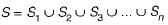For S to be infinite set, atleast one of sets Si must be infinite, since if all Si w ere finite, then S will also be finite.

Test: Set Theory & Algebra- 1 - Question 2

### Let A be a finite set of size n. The number of elements in the power set of A x A is

Detailed Solution for Test: Set Theory & Algebra- 1 - Question 2

Number of elements in A x A = n2
∴ Number of elements in the power set of
A x A =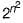Test: Set Theory & Algebra- 1 - Question 3

### The number of elements in the power set P(S) of the set S = {(φ), 1, (2, 3)} is:

Detailed Solution for Test: Set Theory & Algebra- 1 - Question 3

if a set has. n elements then its powers set has 2n elements.
Given set S =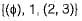Number of elements in S = 3
∴ The number of elements in powerset (S)
= 23
= 8

Test: Set Theory & Algebra- 1 - Question 4

Suppose X and V are sets and |X| and |Y| are their respective cardinalities. It is given that there are exactly 97 functions from X to Y. From this one can conclude that

Detailed Solution for Test: Set Theory & Algebra- 1 - Question 4

X and Y are sets.
The card in a lities of X and Y are |X| and |Y|  respectively.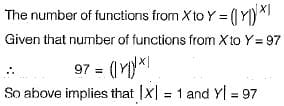Test: Set Theory & Algebra- 1 - Question 5

Suppose A is a finite set with n elements. The number of elements in the Largest equivalence relation of A is

Detailed Solution for Test: Set Theory & Algebra- 1 - Question 5

A is a finite set with n elements. The largest equivalence relation of A is the cross-product A x A and the number of elements in the cross product A x A is n2.

Test: Set Theory & Algebra- 1 - Question 6

The number of functions from an m element set to an n element set is

Detailed Solution for Test: Set Theory & Algebra- 1 - Question 6

The number of functions from an m element set to an n element set is nm.

Test: Set Theory & Algebra- 1 - Question 7

The number of binary relations on a set with n elements is

Detailed Solution for Test: Set Theory & Algebra- 1 - Question 7

The maximum number of elements in a binary relation on a set A with n elements = Number of elements in A x A = n2.
Each element has two choices, either to appear on a binary relation or doesn’t appear on a binary relation.
∴ Number of binary relations =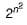Test: Set Theory & Algebra- 1 - Question 8

The binary relation S = f (empty set) on set A = {1,2, 3} is

Detailed Solution for Test: Set Theory & Algebra- 1 - Question 8

The empty relation on any set is always transitive and symmetric but not reflexive.

Test: Set Theory & Algebra- 1 - Question 9

The number of different n x n symmetric matrices with each element being either 0 or 1 is: [Note: power (2, x) is same as 2X]

Detailed Solution for Test: Set Theory & Algebra- 1 - Question 9

In a symmetric matrix, the lower triangle must be the mirror image of upper triangle using the diagonal as mirror. Diagonal elements may be anything. Therefore, when we are counting symmetric matrices we count how many ways are there to fill the upper triangle and diagonal elements. Since the first row has n elements, second (n - 1) elements, third row ( n - 2) elements and so on upto last row, one element.
Total number of elements in diagonal + upper triangle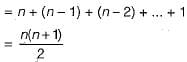Now, each one of these elements can be either 0 or 1. So total number of ways we can fill these elements is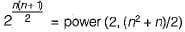Since there is no choice for lower triangleelements the answer is power (2, {n2 + n)/2).

Test: Set Theory & Algebra- 1 - Question 10

The set {1, 2, 3, 5, 7, 8, 9} under multiplication modulo 10 is not a group. Given below are four possible reasons. Which one of them is false?

Detailed Solution for Test: Set Theory & Algebra- 1 - Question 10

Let A = { 1, 2, 3, 5, 7, 8, 9}
Construct the table for any x, y ∈ A such that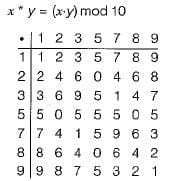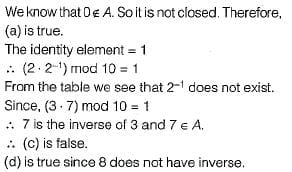## GATE Electrical Engineering (EE) 2024 Mock Test Series

23 docs|285 tests
Information about Test: Set Theory & Algebra- 1 Page
In this test you can find the Exam questions for Test: Set Theory & Algebra- 1 solved & explained in the simplest way possible. Besides giving Questions and answers for Test: Set Theory & Algebra- 1, EduRev gives you an ample number of Online tests for practice

## GATE Electrical Engineering (EE) 2024 Mock Test Series

23 docs|285 tests